# Calculate Area - circles, triangles, rectangles and other figures...

Below you can find an online area calculator for some common shapes. An area is the amount of space covered by a flat surface or piece of land, described as a measurement. There are several well-known formulas for the areas of simple geometric shapes such as circles, triangles and rectangles.

 Using these formulas, the area of any polygon can be found by dividing the polygon into simpler figures, for example, triangles. For shapes with curved edges, calculus is usually required to determine the area. The problem of finding out the area of plane figures, simple or complex, was an important motivation for the historical development of calculus.

### Calculate Area of a CircleThe formula is just A = πr2
If your radius is 1 inch, then the area is 3.1416 square inches.

### Calculate Area of a Triangle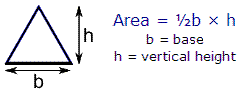The formula is as simple as A = bh/2
If your given base is 3 inches and your vertical height is 8.2 inches, then the area of that given triangle is 12.3 square inches.

 Please enable JavaScript codes to be able to use this calculator.

 Rectangle Width = Height = Area = Parallelogram Base = Height = Area = Triangle Base = Height = Area = Trapezoid Base 1 = Base 2 = Height = Area = Circle Radius = Area = Ellipse Semi-axis a = Semi-axis b = Area =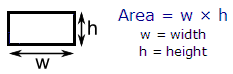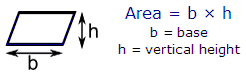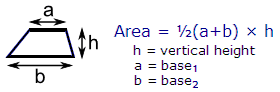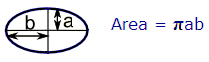From 'Calculate Area' to home

From 'Calculate Area' to Free Online Calculators